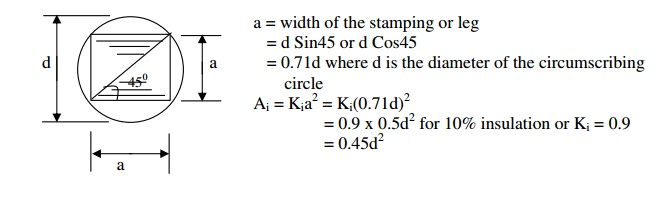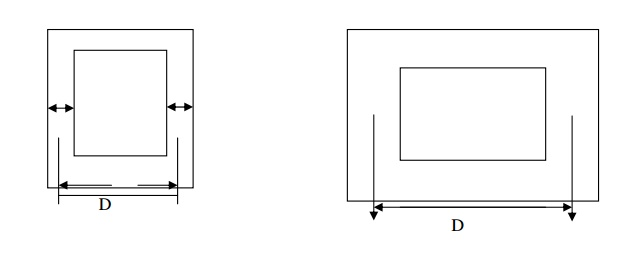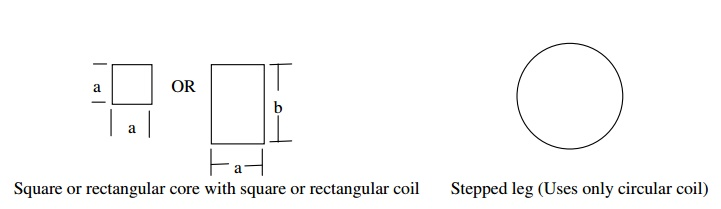Home | | Design of Electrical Machines | Core design - Design of Transformers

# Core design - Design of Transformers

Net iron area of the leg or limb or core Ai. For a given area Ai, different types of core section that are used in practice are circular, rectangular and square.

Core design

Net iron area of the leg or limb or core AiFor a given area Ai, different types of core section that are used in practice are circular, rectangular and  square.It is clear that the rectangular core calls for more length of copper for the same number of turns as compared to circular core. Therefore circular core is preferable to rectangular or square core.Very high values of mechanical forces under short circuit conditions tries to deform the shape of  the square or rectangular coil (the mechanical forces try to deform to a circular shape) and hence damage  the coil and insulation. Since this is not so in case of circular coils, circular coils are preferable to square  or rectangular coils.

Thus a circular core and a circular coil is preferable. Since the core has to be of laminated type,  circular core is not practicable as it calls for more number of different size laminations and poses the  problem of securing them together is in position. However, a circular core can be approximated to a  stepped core having infinite number of steps. Minimum number of steps one and the number of steps in  practice is limited to a definite number. Whenever a stepped core is employed a circular coil is used.## Leg or limb section details: -

The different types of leg sections used are rectangular, square and stepped.

## 1. Rectangular core (with a rectangular coil)a = width of the stamping or leg

b = gross thickness of the assembled core or width of the  transformer

Ai = net iron area of the leg or limb or core

= a xKi b for a core type transformer

Ki = iron factor or stacking factor

2a = width of the central leg

b = width of the transformer

Ai  = 2a x Kib for a shell type transformer

## 2. (a) Square core (with a square coil)a = width of the leg

a = width of the transformer

Ai = Kia2  for a core transformer

2a = width of the central leg

2a = width of the transformer

Ai = Ki (2a)2 for a shell type transformer

## (b) Square core (with a circular coil)a = width of the stamping or leg

= d Sin45 or d Cos45

= 0.71d where d is the diameter of the circumscribing circle

Ai = Kia2  = Ki(0.71d)2

= 0.9 x 0.5d2 for 10% insulation or Ki = 0.9

= 0.45d2

Area of the circumscribing circle Ac = πd2/4 = 0.785d2It is clear that Ai is only 57.3% of Ac. Rest of the area 42.7% of Ac is not being utilized usefully. In order  to utilize the area usefully, more number of steps is used. This leads to 2 stepped, 3 stepped etc core.

## 3. Cruciform or 2-stepped core:

a = width of the largest stamping

b = width of the smallest stamping

Gross area of the core Ag = ab + 2b(a-b)/2 = 2ab-b2

Since a=d Cosθ and b=d SinθIt is clear that addition of one step to a square core, enhances the utilization of more space of the circumscribing circle area.

## 4. Three stepped core:Width of the largest stamping a = 0.9d

Width of the middle stamping b = 0.7d

Width of the smallest stamping c = 0.42d

Ai =0.6d2

## 5. Four stepped core:Note : As the number of steps increases, the diameter of the circumscribing circle reduces. Though the  cost of the core increases, cost of copper and size of the coil or transformer reduces.

### Yoke section details:

The purpose of the yoke is to connect the legs providing a least reluctance path. In order to limit  the iron loss in the yoke, operating flux density is reduced by increasing the yoke area. Generally yoke  area is made 20% more than the leg area..

Note:

1. Whenever the yoke area is different from the leg area, yoke can considered to be of rectangular  type for convenience.

2. In general height of the yoke Hy can be taken as (1.0 to 1.5) a. When there is no data about the  yoke area, consider Hy = a.The different types of yoke sections used are square, rectangular and stepped.

### Window area and core proportionIf Hw = height of the window, Ww = width of the window, then Aw = HwWw

In order to limit the leakage reactance of the transformer, Hw is made more than Ww. In practice Hw / Ww lies between 2.5 and 3.5.Study Material, Lecturing Notes, Assignment, Reference, Wiki description explanation, brief detail
Design of Electrical Machines : Transformers : Core design - Design of Transformers |